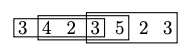# Jay Pantone

## Assistant ProfessorMarquette University jay.pantone@marquette.edu

#### Completing the Structural Analysis of the 2x4 Permutation Classes

with Samuel Miner

We study the structure and enumeration of the final two 2x4 permutation classes, completing a research program that has spanned almost two decades. For both classes, careful structural analysis produces a complicated functional equation. One of these equations is solved with the guess-and-check paradigm, while the other is solved with kernel method-like techniques and Gröbner basis calculations.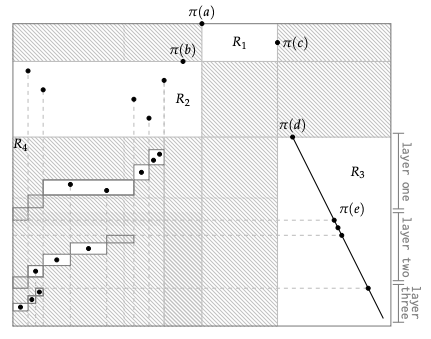#### Universal Layered Permutations

with Michael Albert, Michael Engen, and Vince Vatter
Published in the Electronic Journal of Combinatorics.

We establish an exact formula for the length of the shortest permutation containing all layered permutations of length n, proving a conjecture of Gray.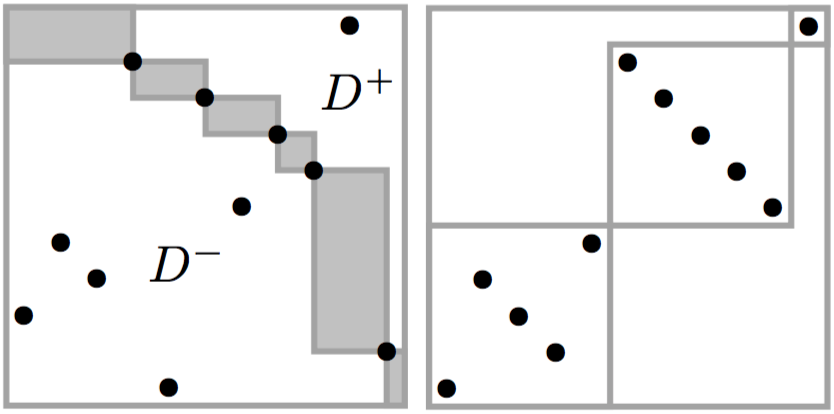#### A Structural Characterisation of $$\operatorname{Av}(1324)$$ and New Bounds on its Growth Rate

with David Bevan, Robert Brignall, and Andrew Elvey Price
Conference Paper — Published in Electronic Notes in Discrete Mathematics.
Full Paper — Submitted.

We establish an improved lower bound of 10.271 for the exponential growth rate of the class of permutations avoiding the pattern $$1324$$, and an improved upper bound of 13.5. These results depend on a new exact structural characterisation of $$1324$$-avoiders as a subclass of an infinite staircase grid class, together with precise asymptotics of a small domino subclass whose enumeration we relate to West-two-stack-sortable permutations and planar maps. The bounds are established by carefully combining copies of the dominoes in particular ways consistent with the structural characterisation. The lower bound depends on concentration results concerning the substructure of a typical domino, the determination of exactly when dominoes can be combined in the fewest distinct ways, and technical analysis of the resulting generating function.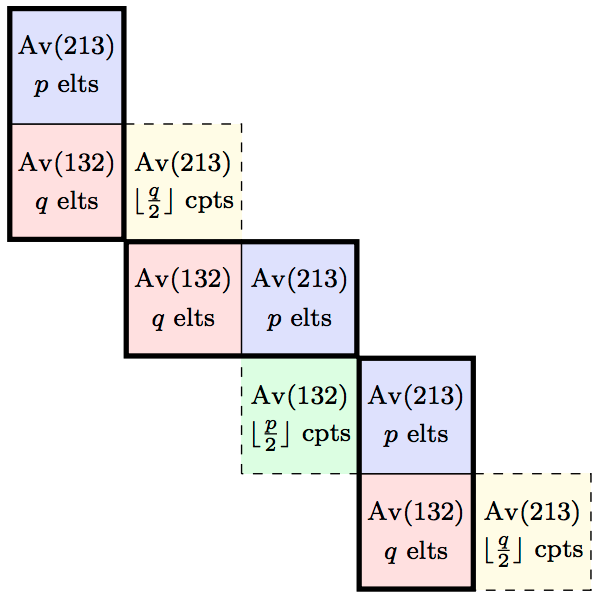#### Shift Equivalence in the Generalized Factor Order

with Jennifer Fidler, Daniel Glasscock, Brian Miceli, and Min Xu
Published in Archiv der Mathematik.

We provide a geometric condition that guarantees strong Wilf equivalence in the generalized factor order. This provides a powerful tool for proving specific and general Wilf equivalence results, and several such examples are given.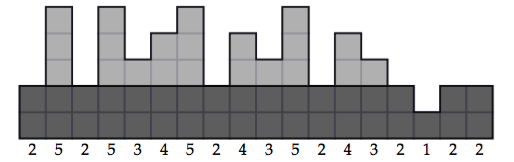#### On the Growth of Merges and Staircases of Permutation Classes

with Michael Albert and Vincent Vatter
To appear in the Rocky Mountain Journal of Mathematics.

There is a well-known upper bound on the growth rate of the merge of two permutation classes. Curiously, there is no known merge for which this bound is not achieved. Using staircases of permutation classes, we provide sufficient conditions for this upper bound to be achieved. In particular, our results apply to all merges of principal permutation classes. We end by demonstrating how our techniques can be used to reprove a result of Bóna.#### Is the Full Susceptibility of the Square-Lattice Ising Model a Differentially Algebraic Function?

with Tony Guttmann, Iwan Jensen, and Jean-Marie Maillard.
Published in the Journal of Physics, A.

We study the class of non-holonomic power series with integer coefficients that reduce, modulo primes, or powers of primes, to algebraic functions. In particular we try to determine whether the susceptibility of the square-lattice Ising model belongs to this class, and more broadly whether the susceptibility is a solution of a differentially algebraic equation.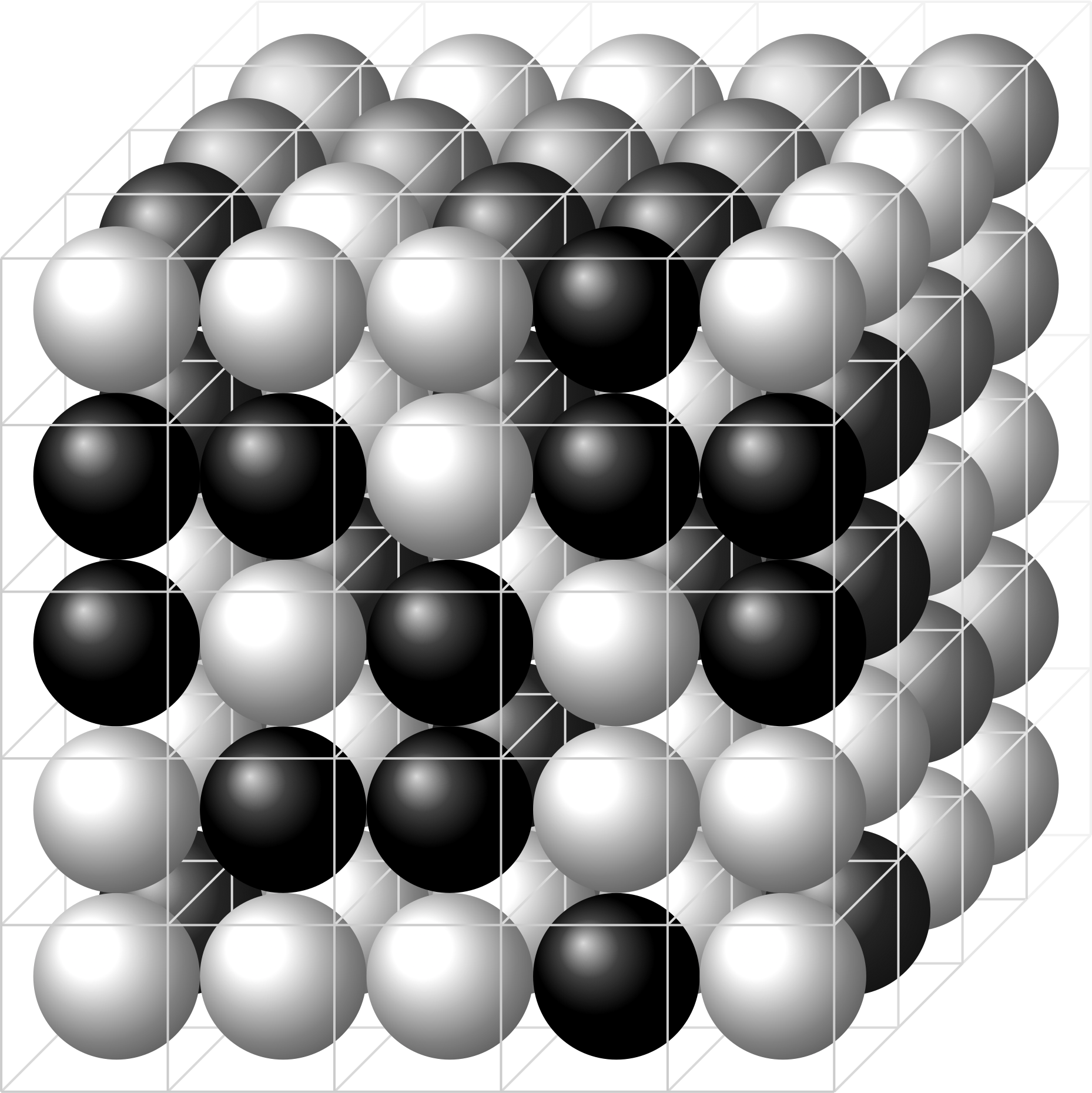credit: Vegar Ottesen [CC BY-SA 3.0], from Wikimedia Commons

#### The Asymptotic Number of Simple Singular Vector Tuples of a Cubical Tensor

Published in the Online Journal of Analytic Combinatorics.

S. Ekhad and D. Zeilberger recently proved that the multivariate generating function for the number of simple singular vector tuples of a generic $$m_1 \times \cdots \times m_d$$ tensor has an elegant rational form involving elementary symmetric functions, and provided a partial conjecture for the asymptotic behavior of the cubical case $$m_1 = \cdots = m_d$$. We prove this conjecture and further identify completely the dominant asymptotic term, including the multiplicative constant. Finally, we use the method of differential approximants to conjecture that the subdominant connective constant effect observed by Ekhad and Zeilberger for a particular case in fact occurs more generally.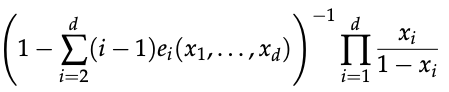#### Growth Rates of Permutation Classes: Categorization up to the Uncountability Threshold

with Vincent Vatter
To appear in the Israel Journal of Mathematics.

In the antecedent paper to this it was established that there is an algebraic number $$\xi\approx 2.30522$$ such that while there are uncountably many growth rates of permutation classes arbitrarily close to $$\xi$$, there are only countably many less than $$\xi$$. Here we provide a complete characterization of the growth rates less than $$\xi$$. In particular, this classification establishes that $$\xi$$ is the least accumulation point from above of growth rates and that all growth rates less than or equal to $$\xi$$ are achieved by finitely based classes. A significant part of this classification is achieved via a reconstruction result for sum indecomposable permutations. We conclude by refuting a suggestion of Klazar, showing that $$\xi$$ is an accumulation point from above of growth rates of finitely based permutation classes.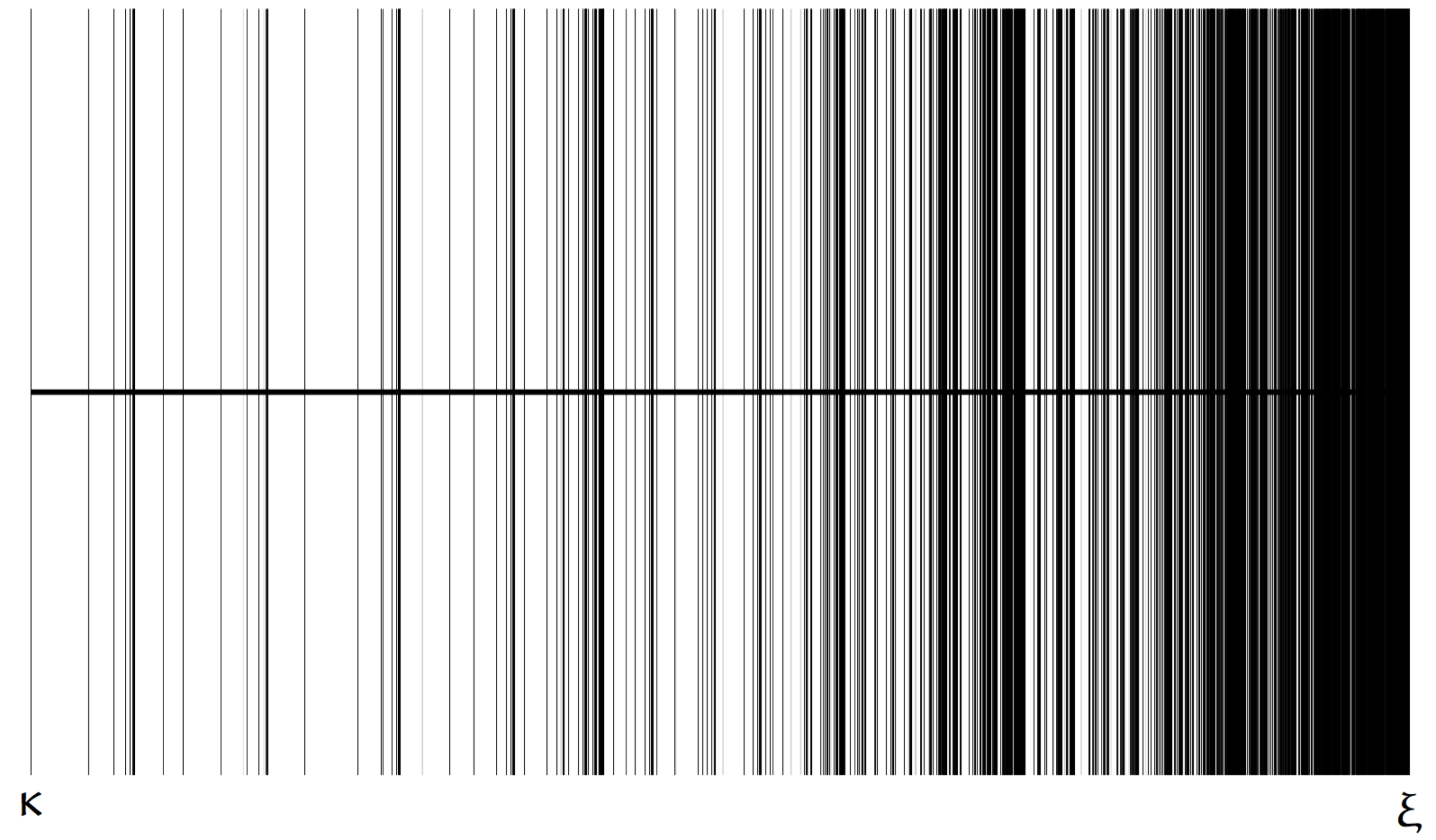#### Pattern Avoidance in Forests of Binary Shrubs

with David Bevan, Derek Levin, Peter Nugent, Lara Pudwell, Manda Riehl and ML Tlachac
Published in Discrete Mathematics and Theoretical Computer Science.

We investigate pattern avoidance in permutations satisfying some additional restrictions. These are naturally considered in terms of avoiding patterns in linear extensions of certain forest-like partially ordered sets, which we call binary shrub forests. In this context, we enumerate forests avoiding patterns of length three. In four of the five non-equivalent cases, we present explicit enumerations by exhibiting bijections with certain lattice paths bounded above by the line $$y=\ell x$$, for some $$\ell\in\mathbb{Q}^+$$, one of these being the celebrated Duchon's club paths with $$\ell=2/3$$. In the remaining case, we use the machinery of analytic combinatorics to determine the minimal polynomial of its generating function, and deduce its growth rate.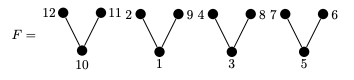#### Generating Permutations with Restricted Containers

with Michael Albert, Cheyne Homberger, Nathaniel Shar, and Vincent Vatter
Published in the Journal of Combinatorial Theory, Series A.

We investigate a generalization of stacks that we call $$\mathcal{C}$$-machines. We show how this viewpoint rapidly leads to functional equations for the classes of permutations that $$\mathcal{C}$$-machines generate, and how these systems of functional equations can frequently be solved by either the kernel method or, much more easily, by guessing and checking. General results about the rationality, algebraicity, and the existence of Wilfian formulas for some classes generated by $$\mathcal{C}$$-machines are given. We also draw attention to some relatively small permutation classes which, although we can generate thousands of terms of their enumerations, seem to not have D-finite generating functions.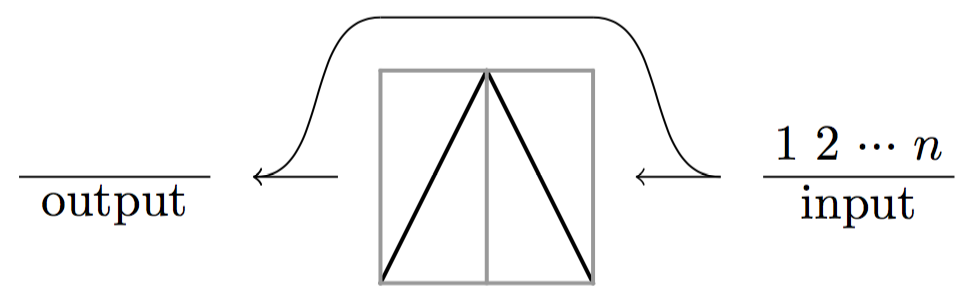#### Pattern-Avoiding Involutions: Exact and Asymptotic Enumeration

with Miklós Bóna, Cheyne Homberger, and Vincent Vatter
Published in the Australasian Journal of Combinatorics.

We consider the enumeration of pattern-avoiding involutions, focusing in particular on sets defined by avoiding a single pattern of length 4. We directly enumerate the involutions avoiding $$1342$$ and the involutions avoiding $$2341$$. As we demonstrate, the numerical data for these problems exhibits some surprising behavior. This strange behavior even provides some very unexpected data related to the number of $$1324$$-avoiding permutations.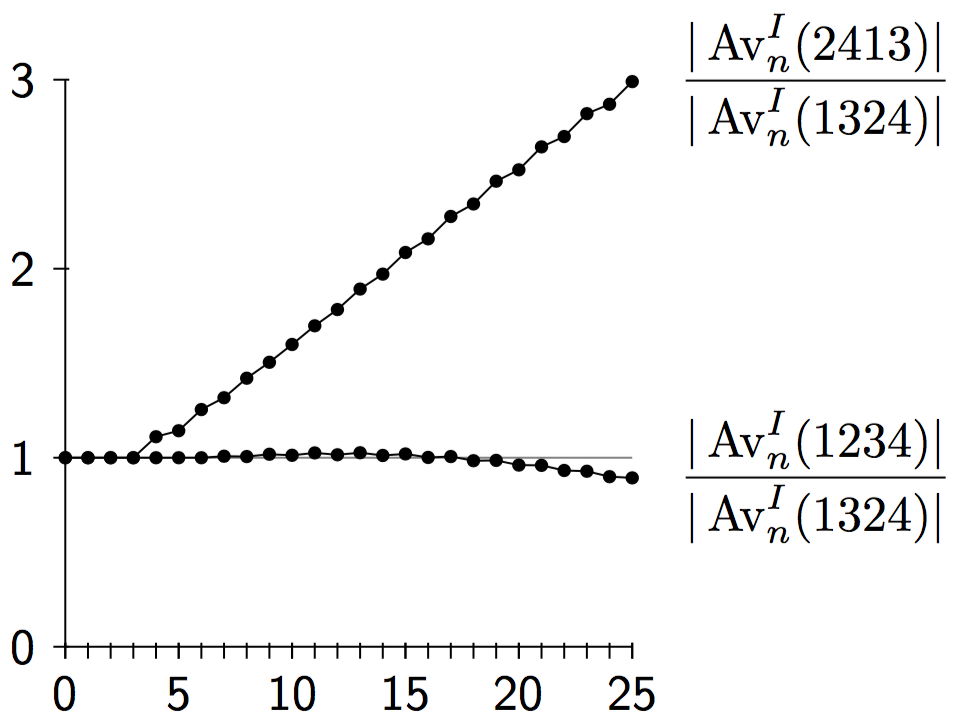#### Deflatability of Permutation Classes

with Michael Albert, Mike Atkinson, and Cheyne Homberger
Published in the Australasian Journal Combinatorics.

A permutation class is said to be deflatable if its simple permutations are contained within a proper subclass. Deflatable classes are often easier to describe, analyze, and enumerate than their non-deflatable counterparts. This paper presents theorems guaranteeing the non-deflatability of principal classes, constructs an infinite family of deflatable principal classes, and provides examples of each.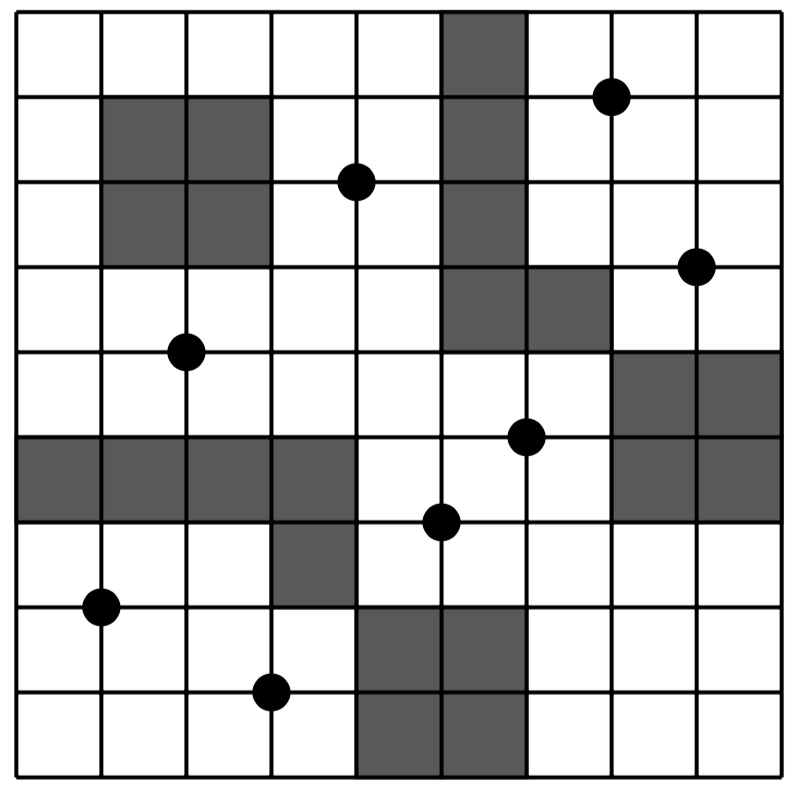#### On Isomorphism Classes of Generalized Fibonacci Cubes

with Jernej Azarija, Sandi Klavžar, Jaehun Lee, and Yoomi Rho
Published in the European Journal of Combinatorics.

The generalized Fibonacci cube $$Q_d(f)$$ is the subgraph of the $$d$$-cube $$Q_d$$ induced on the set of all strings of length $$d$$ that do not contain $$f$$ as a substring. It is proved that if $$Q_d(f) \cong Q_d(f')$$ then $$|f|=|f'|$$. The key tool to prove this result is a result of Guibas and Odlyzko about the autocorrelation polynomial associated to a binary string. It is also proved that there exist pairs of strings $$f, f'$$ such that $$Q_d(f) \cong Q_d(f')$$, where $$|f| \ge \frac{2}{3}(d+1)$$ and $$f'$$ cannot be obtained from $$f$$ by its reversal or binary complementation. Strings $$f$$ and $$f'$$ with $$|f|=|f'|=d-1$$ for which $$Q_d(f) \cong Q_d(f')$$ are characterized.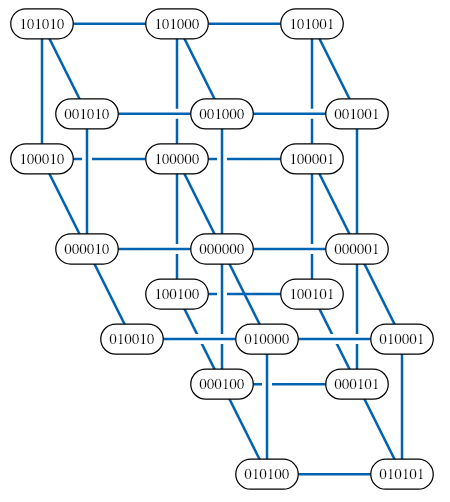credit: David Eppstein [Public domain], from Wikimedia Commons

#### Two Examples of Unbalanced Wilf-Equivalence

with Alex Burstein
Published in the Journal of Combinatorics.

We prove that the set of patterns $$\{1324,3416725\}$$ is Wilf-equivalent to the pattern $$1234$$ and that the set of patterns $$\{2143,3142,246135\}$$ is Wilf-equivalent to the set of patterns $$\{2413,3142\}$$. These are the first known unbalanced Wilf-equivalences for classical patterns between finite sets of patterns.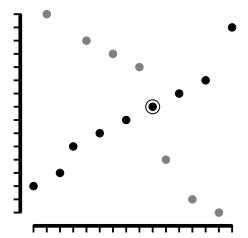#### Equipopularity Classes in the Separable Permutations

with Michael Albert and Cheyne Homberger
Published in the Electronic Journal of Combinatorics.

When two patterns occur equally often in a set of permutations, we say that these patterns are equipopular. Using both structural and analytic tools, we classify the equipopular patterns in the set of separable permutations. In particular, we show that the number of equipopularity classes for length $$n$$ patterns in the separable permutations is equal to the number of partitions of the integer $$n-1$$.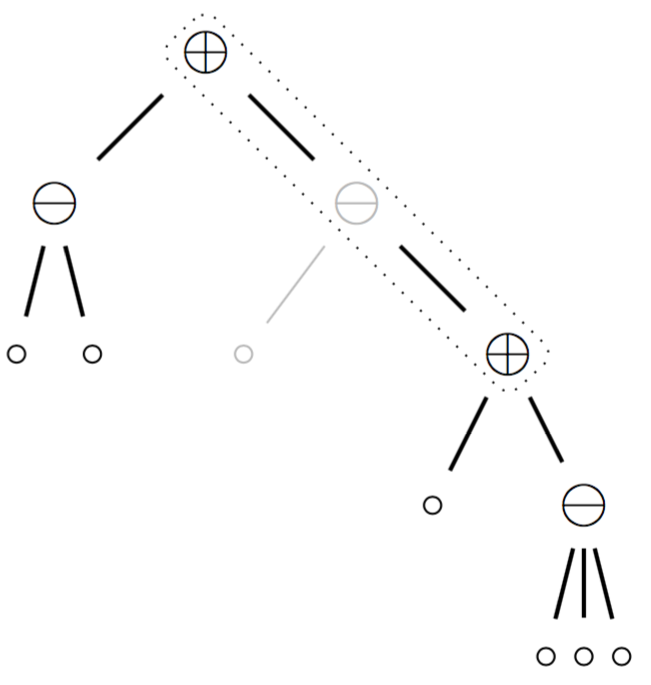#### The Enumeration of Permutations Avoiding 3124 and 4312

Published in the Annals of Combinatorics.

We find the generating function for the class of all permutations that avoid the patterns $$3124$$ and $$4312$$ by showing that it is an inflation of the union of two geometric grid classes.#### On the Rearrangement Conjecture for Generalized Factor Order over $$\mathbb{P}$$.

The Rearrangement Conjecture states that if two words over $$\mathbb{P}$$ are Wilf-equivalent in the factor order on $$\mathbb{P}^\ast$$ then they are rearrangements of each other. We introduce the notion of strong Wilf-equivalence and prove that if two words over $$\mathbb{P}$$ are strongly Wilf-equivalent then they are rearrangements of each other. We further conjecture that Wilf-equivalence implies strong Wilf-equivalence.